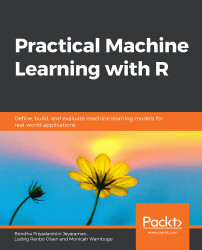•#### Practical Machine Learning with R#### Overview of this book

With huge amounts of data being generated every moment, businesses need applications that apply complex mathematical calculations to data repeatedly and at speed. With machine learning techniques and R, you can easily develop these kinds of applications in an efficient way. Practical Machine Learning with R begins by helping you grasp the basics of machine learning methods, while also highlighting how and why they work. You will understand how to get these algorithms to work in practice, rather than focusing on mathematical derivations. As you progress from one chapter to another, you will gain hands-on experience of building a machine learning solution in R. Next, using R packages such as rpart, random forest, and multiple imputation by chained equations (MICE), you will learn to implement algorithms including neural net classifier, decision trees, and linear and non-linear regression. As you progress through the book, you’ll delve into various machine learning techniques for both supervised and unsupervised learning approaches. In addition to this, you’ll gain insights into partitioning the datasets and mechanisms to evaluate the results from each model and be able to compare them. By the end of this book, you will have gained expertise in solving your business problems, starting by forming a good problem statement, selecting the most appropriate model to solve your problem, and then ensuring that you do not overtrain it.Free Chapter
An Introduction to Machine LearningData Cleaning and Pre-processingFeature EngineeringIntroduction to neuralnet and Evaluation MethodsLinear and Logistic Regression ModelsUnsupervised LearningAppendix## Overview of Unsupervised Learning (Clustering)

Unsupervised learning is a subcategory of machine learning that learns or trains using unlabeled data. In other words, as opposed to supervised learning, where the model is expected to predict or categorize data into a set of known classes, unsupervised learning establishes the structure within data to create the categories or groups.

Before delving into unsupervised learning and, specifically, clustering, there are a few questions you need to answer:

• Based on domain knowledge, does your dataset inherently have subgroups? If yes, how do you identify the subgroups? How many subgroups are present in the dataset?
• Are the members of each subgroup similar? Typically, clustering should only be applied to datasets that have subgroups with somewhat similar datapoints.
• Are there outliers in the dataset? Outliers can often influence the choice of which clustering algorithm to use.

Answering these questions will help us to create a better...